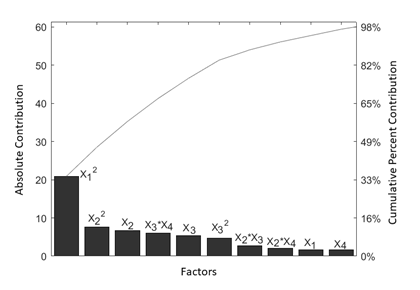Indian Chemical Society

## AbstractPareto plot representing Effects of Factors on 2Mp removal

In this study, removal data of 2-methylpyridine (2Mp) using exfoliated graphene oxide nanocomposite (GON) was modeled by response surface methodology using Doehlert design (DD) matrix. DD involves lesser experimental runs than the other statistical designs, while predicting outputs with relatively low error. The individual and combined effects of parameters namely: temperature, GON dosage, pH levels, and initial 2Mp concentration were explored using a 4-Factor DD. The model developed from statistical analysis suggested a strong relation between pH and 2Mp removal efficiency, with a maximum removal observed at a pH 7.0. From the range of values of the factors chosen for analysis, DD predicted pH 7.10, GON dosage = 1.84 g/L, initial 2Mp loading = 12.77 mg/L and temperature = 26.36ºC as optimal conditions for a removal percentage of 73.51%. The model showed high coefficient of determination (R2) = 0.9906 and the predicted error was found to be less than 2% for prediction of 2Mp removal.

## Keyword

Doehlert design of experiments, Response surface method (RSM), pyridine derivative, graphene oxide (GO) nanomaterial, parameter optimization